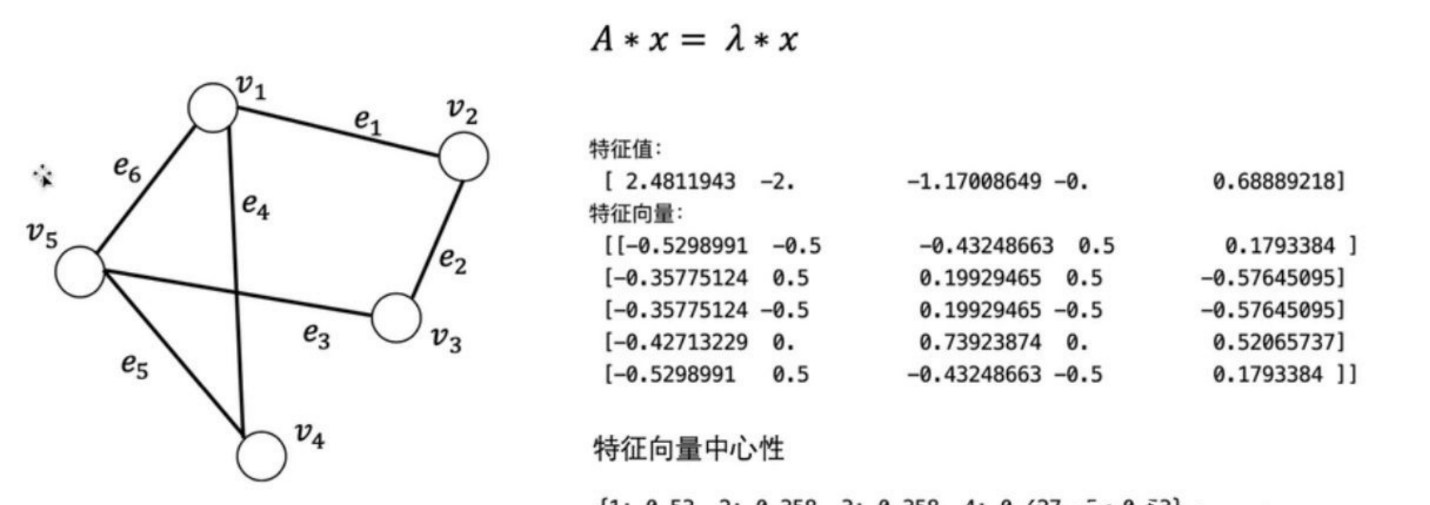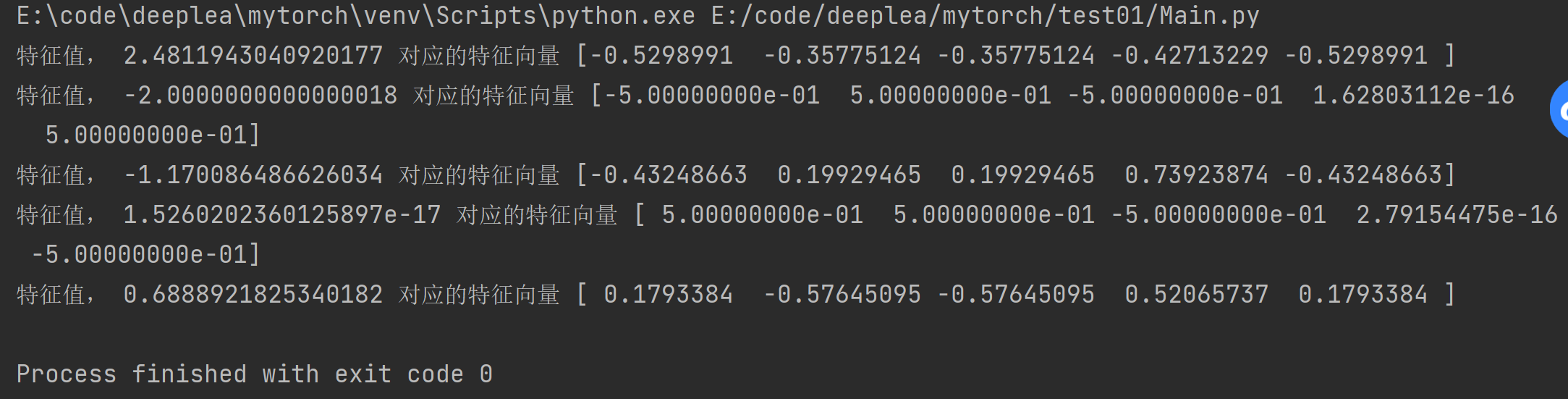• 如何计算特征向量
千次阅读
2020-12-11 05:35:25

TL / DR：尝试nx.eigenvector_centrality_numpy.

这是正在发生的事情：nx.eigenvector_centrality依赖于功率迭代.它所采取的动作相当于将矢量重复乘以相同的矩阵(然后对结果进行归一化).这通常会收敛到最大的特征向量.然而,当存在具有相同(最大)幅度的多个特征值时,它失败.

您的图表是星图.星图有多个“最大”特征值.对于只有两个“外围节点”的星型,您可以轻松检查sqrt(2)和-sqrt(2)是否都是特征值.更一般地,sqrt(N)和-sqrt(N)都是特征值,而其他特征值具有较小的幅度.我相信,对于任何二分网络,这都会发生,标准算法将失败.

数学原因是在n轮迭代之后,解决方案看起来像是c_i lambda_i ^ n v_i / K_n的总和,其中c_i是取决于初始猜测的常数,lambda_i是第i个本征值,v_i是其本征向量, K是归一化因子(适用于总和中的所有项).当存在显性特征值时,lambda_i ^ n / K_n对于主要特征值变为非零常数而对于其他特征值变为0.

但是在你的情况下,你有两个同样大的特征值.较小特征值的贡献仍然为零.但是你留下了(c_1 lambda_1 ^ n v_1 c_2 lambda_2 ^ n v_2)/ K_n.这不会收敛,因为lambda_2是负数.每次迭代时,都会从添加一些v_2的倍数到减去该倍数.

因此,networkx使用的简单特征值中心将不起作用.您可以改为使用nx.eigenvector_centrality_numpy以便使用numpy.

注意：通过快速浏览文档,我不是100％肯定numpy算法保证是最大(正)特征值.它使用numpy算法来寻找特征向量,但我没有在文档中看到它保证它是主要的特征向量.大多数用于查找单个特征向量的算法都会产生显性特征向量,因此您可能没问题.

我们可以添加一个检查：

>只要nx.eigenvector_centrality_numpy返回所有正值,Perron-Frobenius定理就保证这对应于最大的特征值.>如果有些是零,那么肯定会有点棘手,>如果有些是负的,那么它不是主要的特征向量.

更多相关内容
• 本文主要讲解利用Eigen库计算矩阵的特征值及特征向量并与Matlab计算结果进行比较。 C++Eigen库代码 #include #include #include using namespace Eigen; using namespace std; void Eig() { Matrix3d A; A <...
• //计算节点的特征向量 private double[] normalize(double[][] matrix) { int row = matrix.Length; int column = matrix.Length; double[] Sum_column = new double[colu...
//计算节点的特征向量
private double[] normalize(double[][] matrix)
{
int row = matrix.Length;
int column = matrix.Length;
double[] Sum_column = new double[column];
double[] w = new double[row];

if (normalizeType == "和法")
{
for (int i = 0; i < column; i++)
{
Sum_column[i] = 0;
for (int j = 0; j < row; j++)
{
Sum_column[i] += matrix[j][i];
}
}

//进行归一化,计算特征向量W

for (int i = 0; i < row; i++)
{
w[i] = 0;
for (int j = 0; j < column; j++)
{
w[i] += matrix[i][j] / Sum_column[j];
}
w[i] /= row;
}
}

if (normalizeType == "根法")
{
for (int i = 0; i < column; i++)
{
Sum_column[i] = 0;
for (int j = 0; j < row; j++)
{
Sum_column[i] += matrix[j][i];
}
}

//进行归一化,计算特征向量W
double sum = 0;
for (int i = 0; i < row; i++)
{
w[i] = 1;
for (int j = 0; j < column; j++)
{
w[i] *= matrix[i][j] / Sum_column[j];
}

w[i]=Math.Pow(w[i],1.0/row);
sum += w[i];
}

for (int i = 0; i < row; i++)
{
w[i] /= sum;
}
}

if (normalizeType == "幂法")
{
double[] w0 = new double[row];
for (int i = 0; i < row; i++)
{
w0[i] = 1.0/row;
}

//一般向量W（k+1）
double[] w1 = new double[row];
//W（k+1）的归一化向量
double sum = 1.0;
double d = 1.0;
double delt = 0.00001;
while (d > delt)
{
d=0.0;
sum = 0;

//获取向量
for (int j = 0; j < row; j++)
{
w1[j] = 0;
for (int k = 0; k < row; k++)
{
w1[j] += matrix[j][k] * w0[k];
}
sum += w1[j];
}

//向量归一化
for (int k = 0; k < row; k++)
{
w[k] = w1[k] / sum;
d = Math.Max(Math.Abs(w[k] - w0[k]), d);//最大差值
w0[k] = w[k];//用于下次迭代使用
}
}
}
return w;
}
展开全文• torch.tensor([[1,2,3],[4,5,6]]) a,b = np.linalg.eig(x) for i in range (len(a)): print('特征值，',a[i],'对应的特征向量',b[:,i]) 特征值， 2.4811943040920177 对应的特征向量 [-0.5298991 -0.35775124 -0....import  numpy as np
import torch as torch

# 0 1 0 1 1
# 1 0 1 0 0
# 0 1 0 0 1
# 1 0 0 0 1
# 1 0 1 1 0
x=np.array([[0 ,1 ,0 ,1, 1],
[1 ,0, 1, 0, 0],[0, 1, 0, 0, 1],[1, 0, 0, 0, 1],[1, 0, 1, 1, 0]])
# a = torch.tensor([[1,2,3],[4,5,6]])
a,b = np.linalg.eig(x)
for i in range (len(a)):
print('特征值，',a[i],'对应的特征向量',b[:,i])

特征值， 2.4811943040920177 对应的特征向量 [-0.5298991  -0.35775124 -0.35775124 -0.42713229 -0.5298991 ]
特征值， -2.0000000000000018 对应的特征向量 [-5.00000000e-01  5.00000000e-01 -5.00000000e-01  1.62803112e-16
5.00000000e-01]
特征值， -1.170086486626034 对应的特征向量 [-0.43248663  0.19929465  0.19929465  0.73923874 -0.43248663]
特征值， 1.5260202360125897e-17 对应的特征向量 [ 5.00000000e-01  5.00000000e-01 -5.00000000e-01  2.79154475e-16
-5.00000000e-01]
特征值， 0.6888921825340182 对应的特征向量 [ 0.1793384  -0.57645095 -0.57645095  0.52065737  0.1793384 ]展开全文• 在使用AHP(层次分析法)时，需要计算特征值，使用pyhon读取Excel数据处理 此程序使用python读excel数据计算特征值和特征向量； 使用了numpy、xlrd、xlwt库；
• 最近因为课题需要，使用欧式距离来计算多个特征向量间的距离。开始的想法是使用循环来解决，发现计算复杂度高，时间长 在博客中看到作者GoHowz 和其引用frankzd博客，通过矩阵的方法来代替之前循环计算方法，速度...

最近因为课题需要，使用欧式距离来计算多个特征向量间的距离。开始的想法是使用循环来解决，发现计算复杂度高，时间长

在博客中看到作者GoHowz 和其引用frankzd博客，通过矩阵的方法来代替之前循环计算方法，速度提升很多！！！

为了方便后面查询，粘贴了GoHowz 博客中的计算方法如下：

def euclidean_dist(x, y):
"""
Args:
x: pytorch Variable, with shape [m, d]
y: pytorch Variable, with shape [n, d]
Returns:
dist: pytorch Variable, with shape [m, n]
"""

m, n = x.size(0), y.size(0)
# xx经过pow()方法对每单个数据进行二次方操作后，在axis=1 方向（横向，就是第一列向最后一列的方向）加和，此时xx的shape为(m, 1)，经过expand()方法，扩展n-1次，此时xx的shape为(m, n)
xx = torch.pow(x, 2).sum(1, keepdim=True).expand(m, n)
# yy会在最后进行转置的操作
yy = torch.pow(y, 2).sum(1, keepdim=True).expand(n, m).t()
dist = xx + yy
# torch.addmm(beta=1, input, alpha=1, mat1, mat2, out=None)，这行表示的意思是dist - 2 * x * yT
dist.addmm_(1, -2, x, y.t())
# clamp()函数可以限定dist内元素的最大最小范围，dist最后开方，得到样本之间的距离矩阵
dist = dist.clamp(min=1e-12).sqrt()  # for numerical stability
return dist

展开全文• 特征值分析matlab程序，包括左右特征向量及参与因子计算
• 点击蓝字可关注特征值和特征向量计算 特征值和特征向量的几何意义 特征值和特征向量确实有很明确的几何意义，矩阵(既然讨论特征向量的问题，当然是方阵，这里不讨论广义特征向量的概念，就是一般的特征向量)乘以一...matlab 向量
• 文章目录层次分析法计算特征向量归一化前为负的解决方案 层次分析法计算特征向量归一化前为负的解决方案 在进行层次分析法AHP的过程中，我们可能会遇到负特征向量的情况，查了好久，解决方案就是归一化 v=max_x./sum...
• 这是我在数学建模大赛培训时编的程序，有注解，方便大家看的明白，希望对其他参赛选手有所帮助
• 文章目录numpy计算矩阵特征值，特征向量一、示例：二、numpy实现： numpy计算矩阵特征值，特征向量 一、示例： 首先借参考百度demo的来看一下矩阵的特征值和特征向量的解题过程及结果。 可知矩阵A：特征值为1对应的...python
• 《矩阵特征值与特征向量的定义与性质》教学设计.pdf
• Jacobi方法求解特征值特征向量，Jacobi方法求解特征值特征向量MATLAB
• SVD也是对矩阵进行分解，但是和特征分解不同，SVD并不要求要分解的矩阵为方阵。假设我们的矩阵A是一个m×n的矩阵，那么我们定义矩阵A的SVD为：A=UΣVT其中U是一个m×m的矩阵，Σ是一个m×n的矩阵，除了主对角线上的...
• 在MATLAB语言中，求矩阵的特征值和特征向量需要用到两个函数：eig()、diag() diag():可生成一个对角矩阵 调用eig函数的格式为： [x,y]=eig(A) 其中矩阵y的对角线元素存储的是A的所有特征值，且从小到大排列；而...matlab
• 特征值和特征向量计算器为4X4的实矩阵λ 是 [A] 矩阵的特征值 (标量)，如果有一个非零向量 (v) 这样满足以下关系:[A](v) = λ (v)每个向量 (v) 满足这个方程叫做 [A] 属于特征值 λ 的特征向量。作为一个例子，在一...
• 博主：unixfy； 来源：cppblog（个人博客）；...特征向量相似度和距离的计算 相似度： .夹角余弦 ·相关系数 ·Dice ·Jaccard 距离 ·明氏距离 ·欧氏距离 ·马氏距离 ·Jffreys & Matusita 距离 ·Mah...
• 成立，那么，这样的数λ称为矩阵A的特征值，非零向量x称为A的对应于特征值λ的特征向量（1）式还可以写为： （2） 如果想求出矩阵对应的特征值和特征向量就是求式（2）的解了 2.特征值和特征向量的相关函数 （1...matlab
• 计算矩阵R的特征向量和特征矩阵 c = np.linalg.eig(R) 其中特征值为c 特征向量为c import numpy as np # w1为列向量 x11 = np.array([[-3 / 4, -1 / 4, -1 / 8]]).T x12 = np.array([[5 / 4, -1 / 4, -1...python
• 在MATLAB中，计算矩阵A的特征值和特征向量的函数是eig(A)，常用的调用格式有5种：(1) E=eig(A)：求矩阵A的全部特征值，构成向量E。想求最大特征值用：max(eig(A))就好了。(2) [V,D]=eig(A)：求矩阵A的全部特征值，...
• 数值计算方法课件：第三章 矩阵特征值与特征向量计算.ppt
• 今天小编就为大家分享一篇numpy.linalg.eig() 计算矩阵特征向量方式，具有很好的参考价值，希望对大家有所帮助。一起跟随小编过来看看吧
• 1.矩阵特征值和特征向量定义 A为n阶矩阵，若数λ和n维非0列向量x满足Ax=λx，那么数λ称为A的特征值，x称为A的对应于特征值λ的特征向量。式Ax=λx也可写成( A-λ... 计算：A的特征值和特征向量计算行列式得化简...
• 【OpenCV4】计算对称矩阵特征值和特征向量 cv::eigen() 用法详解和代码示例（c++）opencv 计算机视觉
• 本代码用C语言进行编程，可以计算矩阵的特征值和相应的特征向量
• 矩阵的相似定义 相似矩阵的性质 特征值和特征向量的定义...